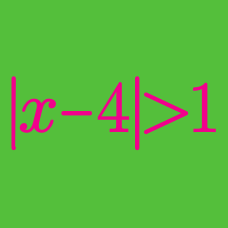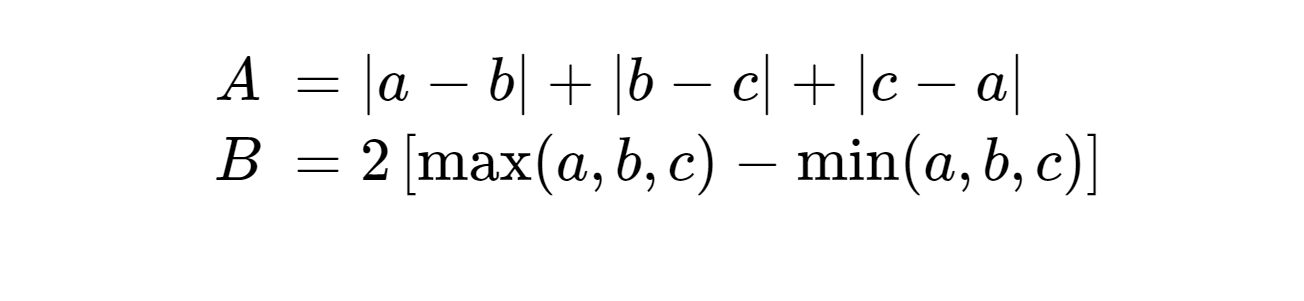Algebra

# Absolute Value Inequalities - Problem SolvingFor all ordered triples of real values $(a, b, c)$, which of these numbers is larger?

If $x, y, z$ are non-zero real, what is the range of

$f(x, y, z) = \frac{ |x+y| } { |x| + |y| } + \frac{ |y+z| } { |y| + |z| } + \frac{ |z+x| } { |z| + |x| } ?$

Consider all monic polynomials $f(x) = x^2 + bx + c$, where $b$ and $c$ are real numbers. What is the minimum value of $N$, where

$N = \max_{x \in [-22, 50]} \vert f(x) \vert?$

Consider all monic polynomials of the form $f_a(x) = x + a$. As $a$ ranges over all real numbers, what is the minimum value of $N_a$, where

$N_a = \max_{x \in [-13, 39] } \lvert f_a(x) \rvert ?$

If the solution to the inequality $\lvert x+ a \rvert \leq 8$ is $7 \leq x \leq 23,$ what is $a?$

×